# 基本元器件 - 运算放大器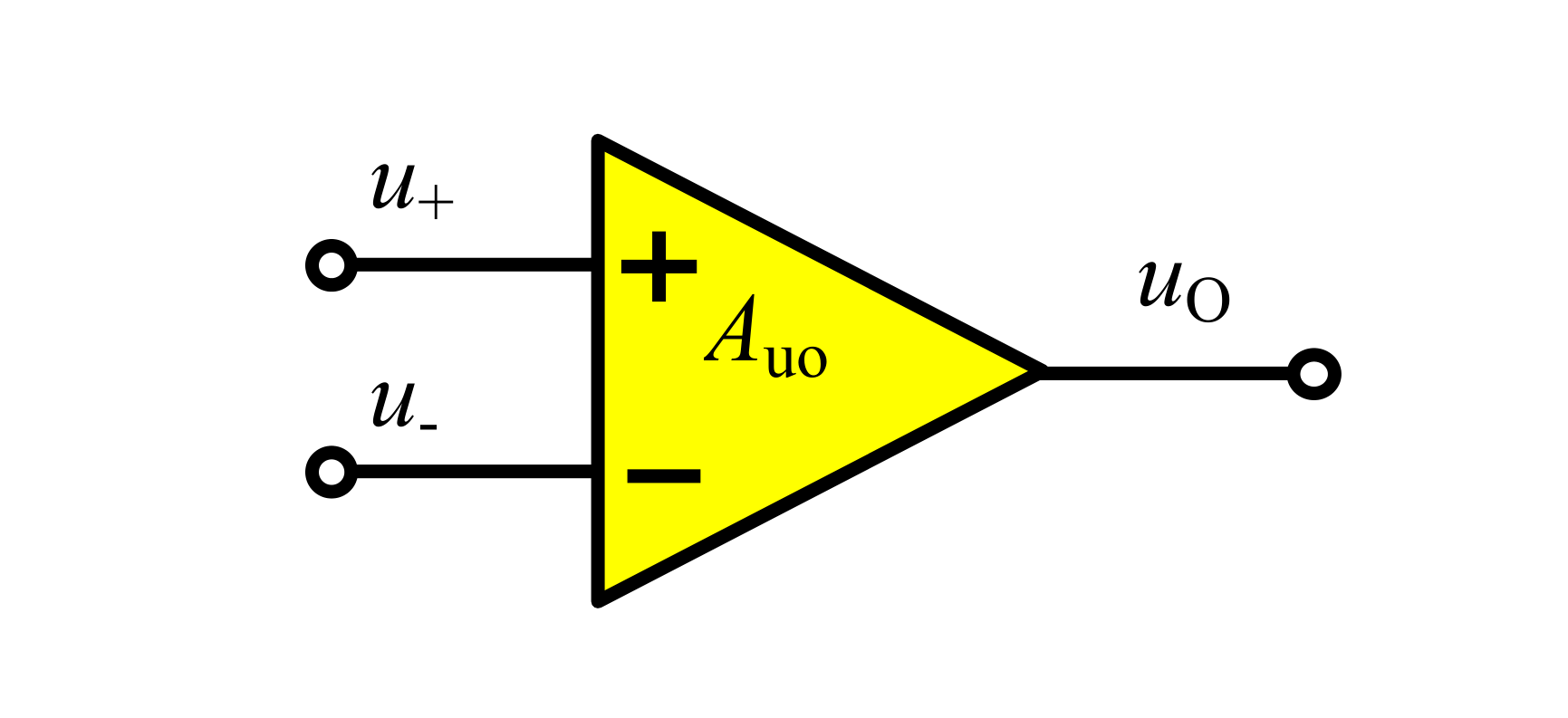$u_o=A_{uo}(u_+-u_-)$

## 运放的工作状态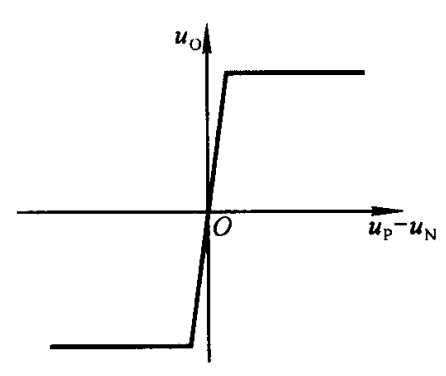• 工作在线性放大区：斜线的斜率为开环电压增益。
• 工作在非线性区：即饱和状态，在图中是左右两端的水平线，输出电压为 $$-U_{om}$$（负电源端子 $$V_-$$ 的电压），或 $$+U_{om}$$（等同于正电源端子 $$V_+$$ 的电压）。

## 运放的虚短与虚断

### 虚短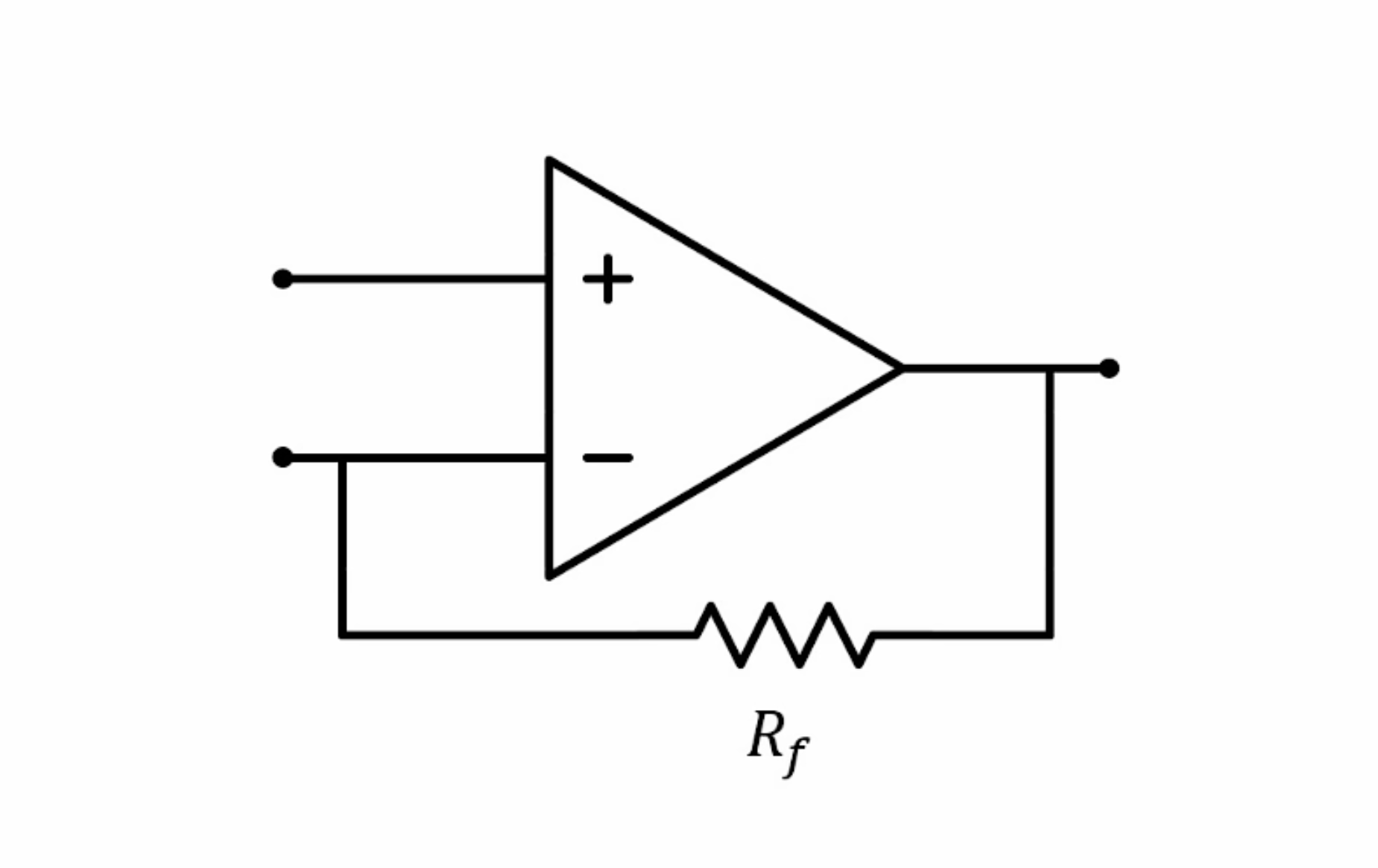## 常用运放电路

### 电压跟随器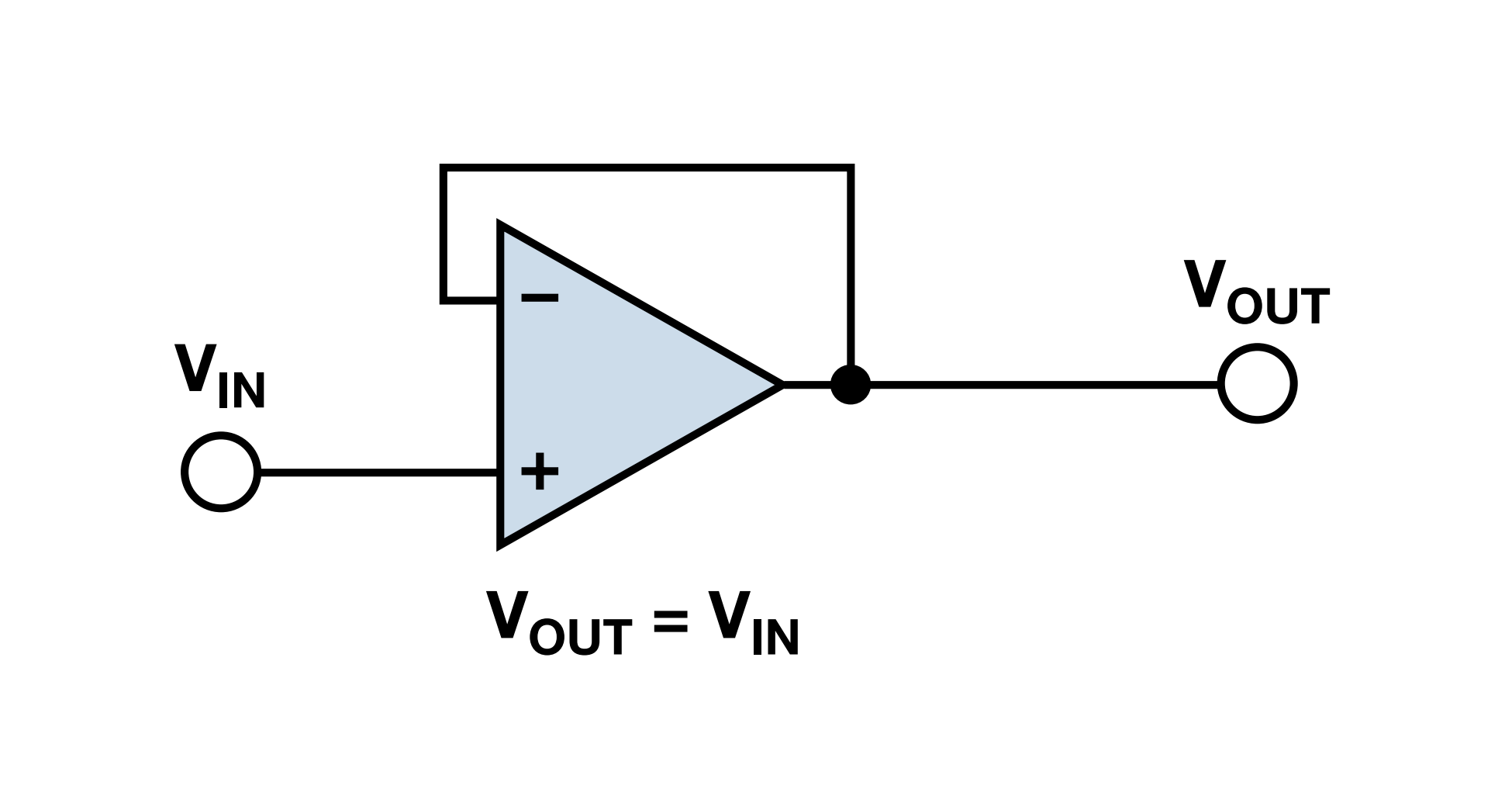### 同相放大器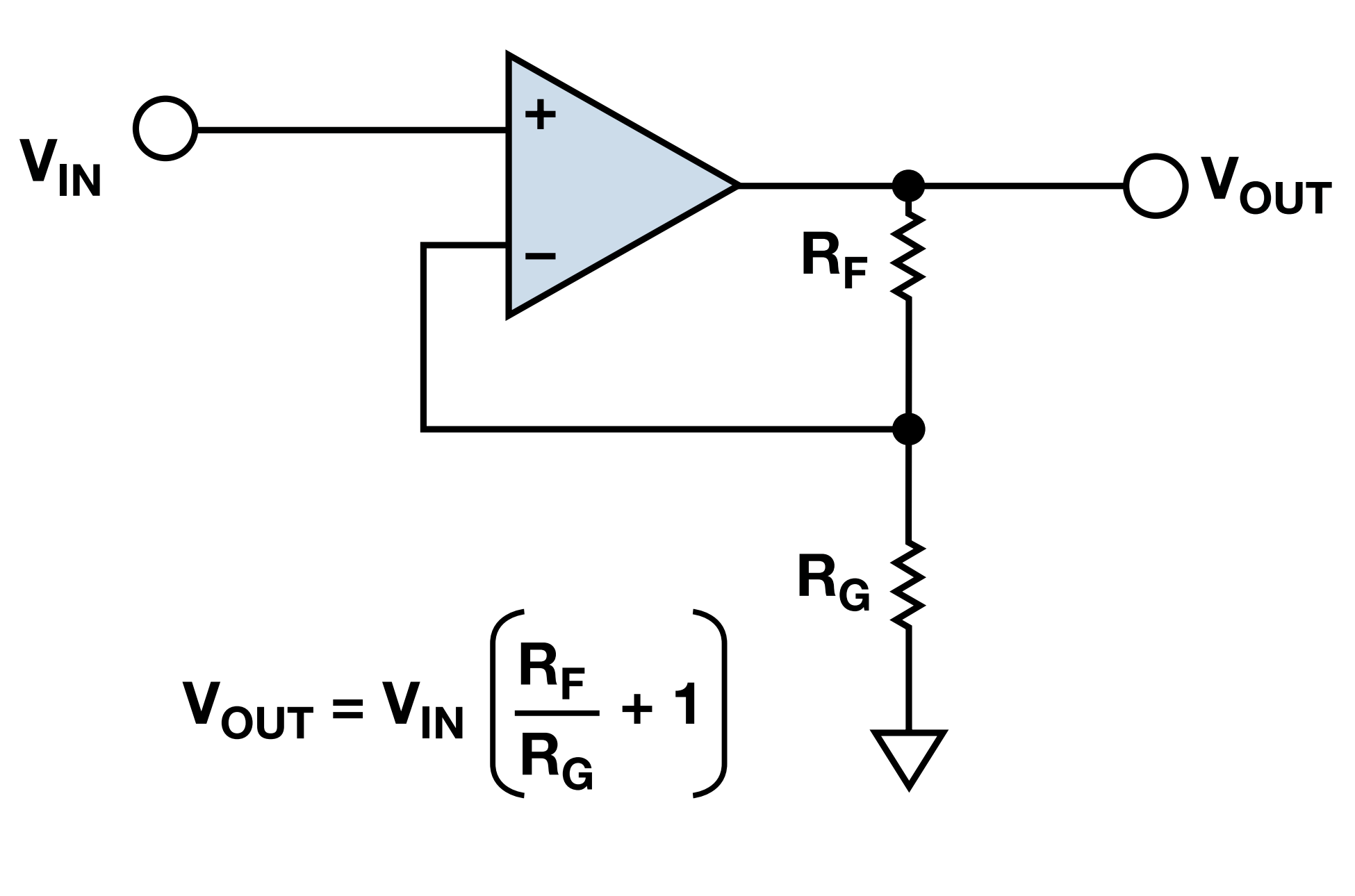1. 因为虚短，所以 $$V_- = V_{IN}$$
2. 因为续断，所以 $$V_-$$ 端输入电流可忽略不计，所以 $$I_{R_G}=I_{R_F}$$，根据欧姆定律，$$\frac{0–V_-}{R_G}=\frac{V_- - V_{OUT}}{R_F}$$，得出 $$V_{OUT}=V_{IN}(\frac{R_F}{R_G}+1)$$

### 反相放大器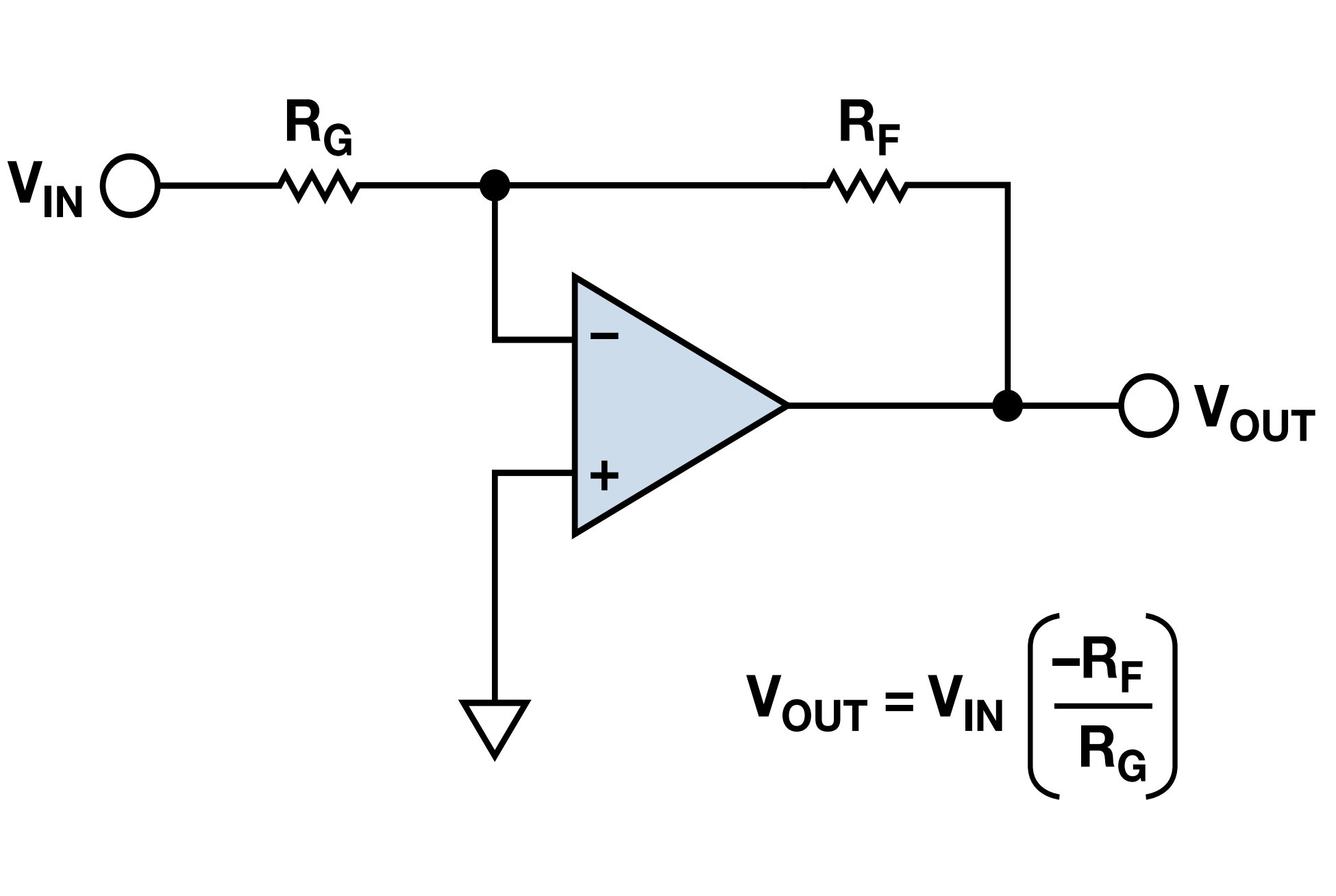### 电压减法器 / 差动放大器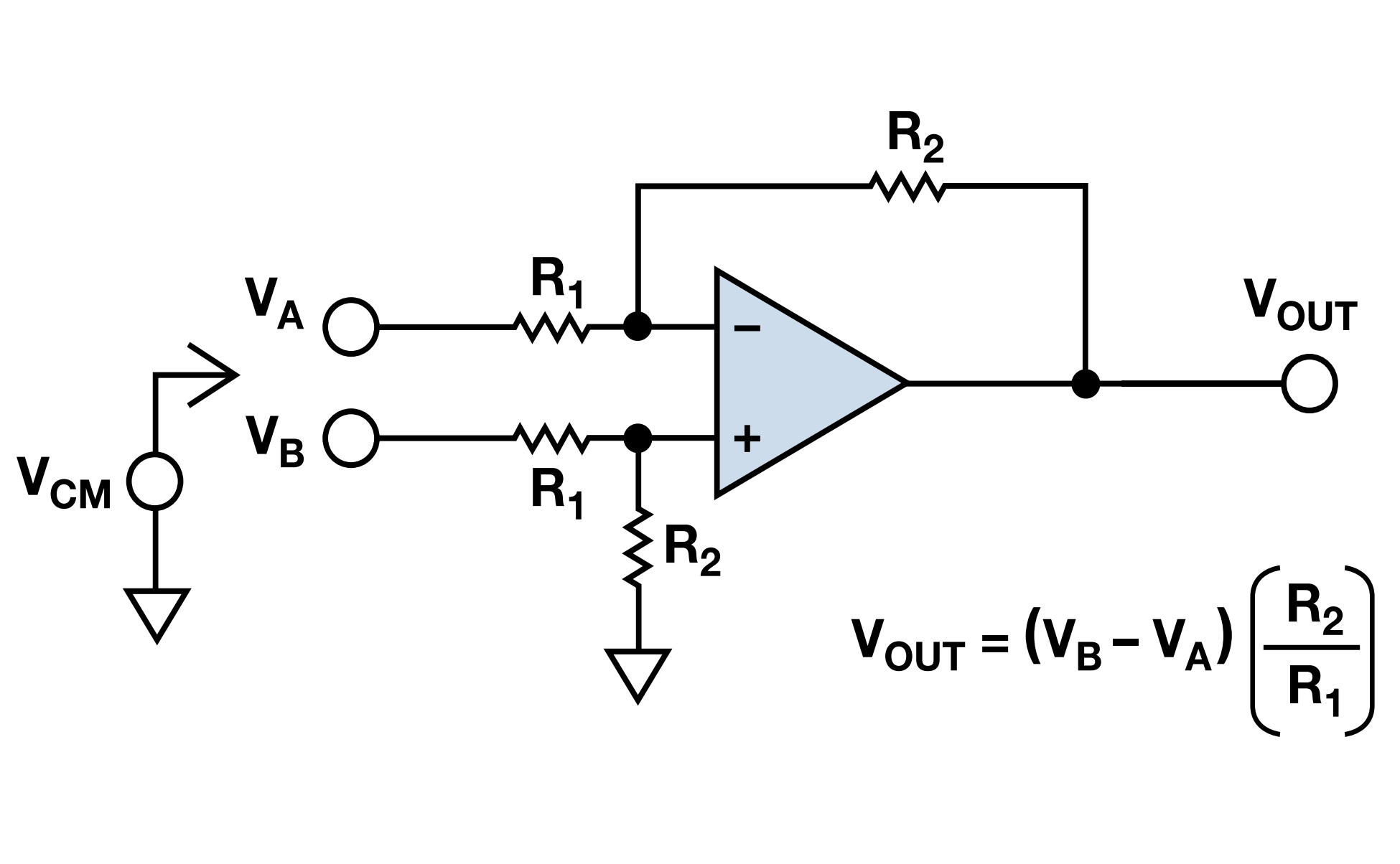### 电压加法器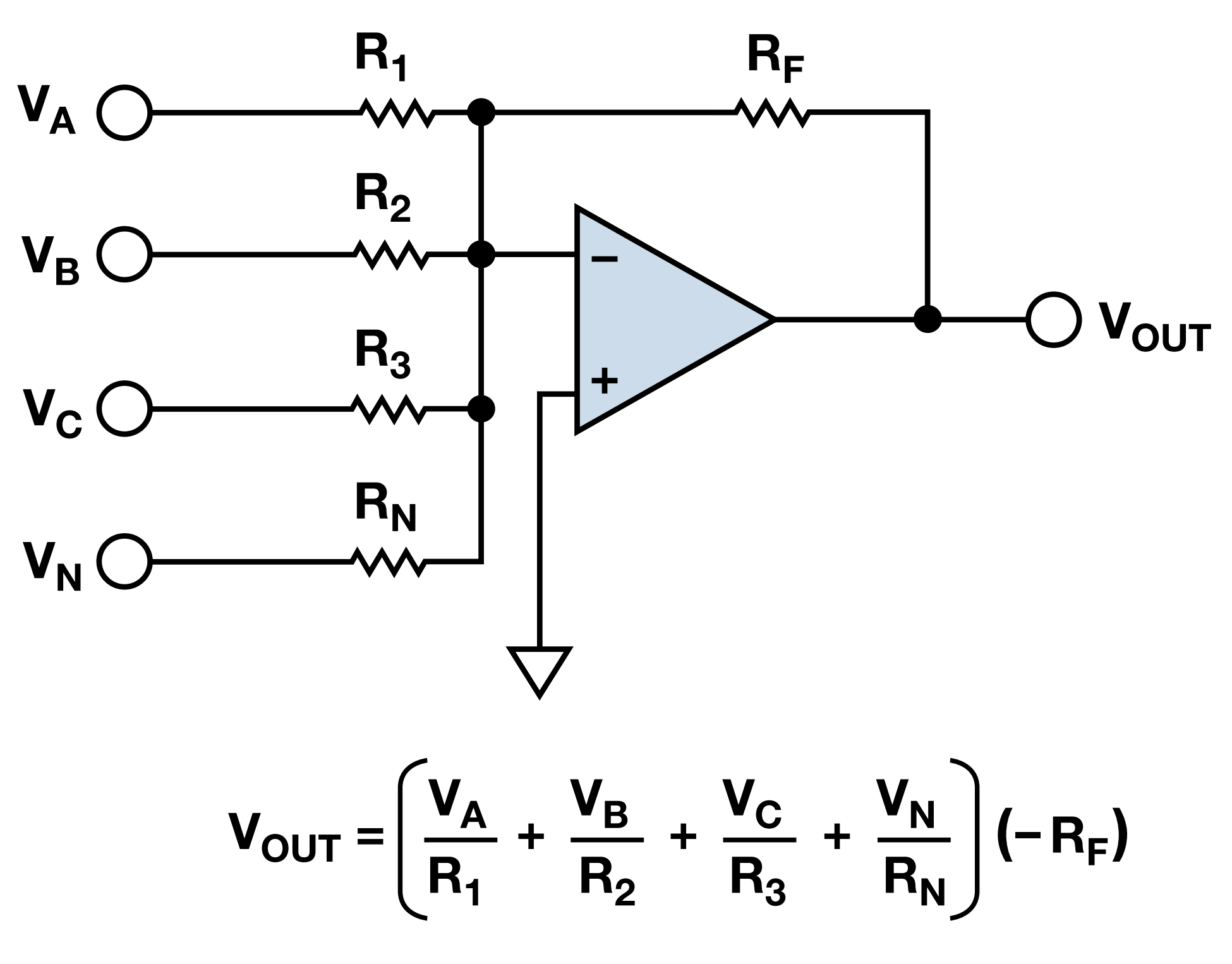### 低通滤波器 / 积分器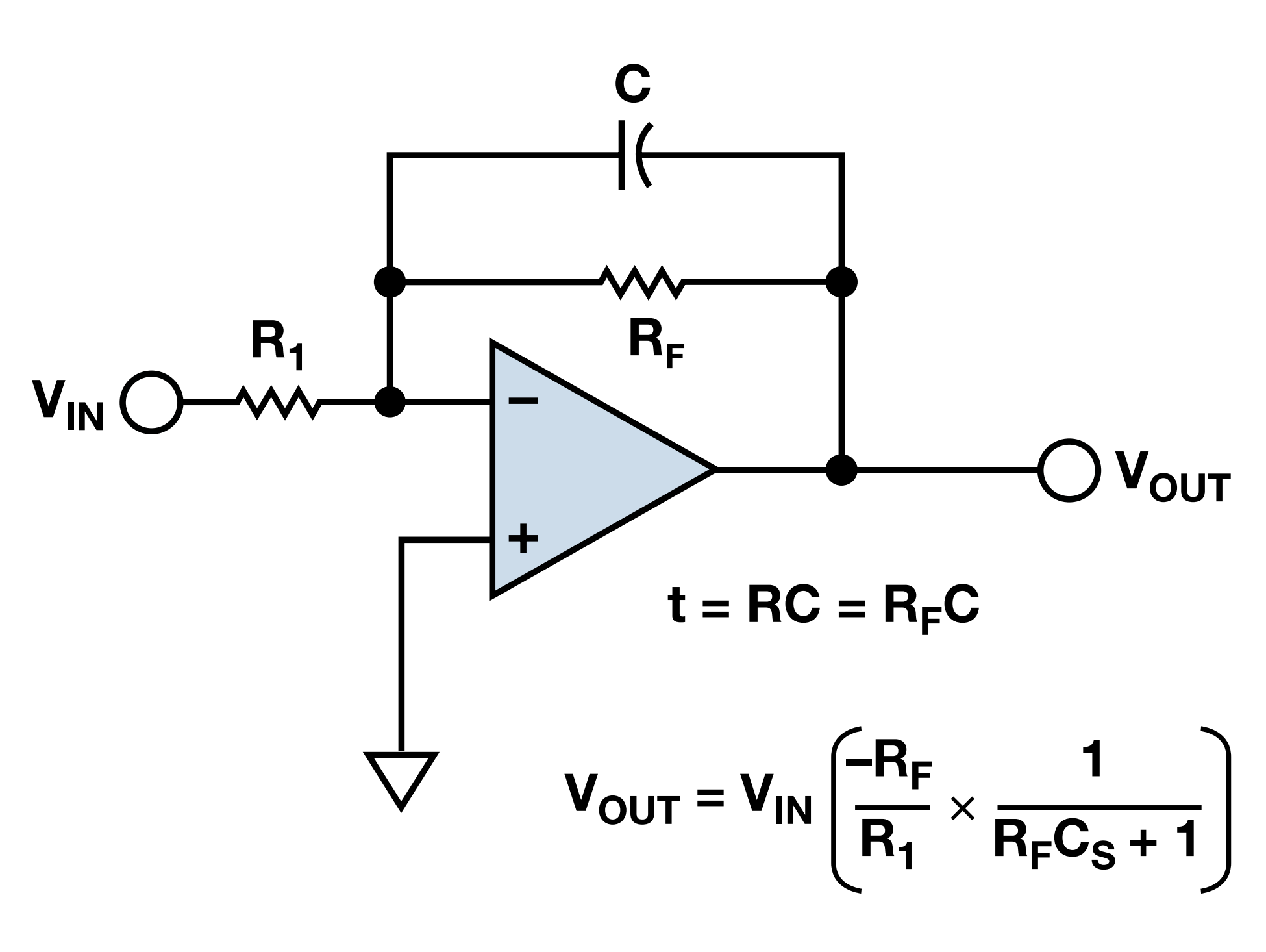### 高通滤波器 / 微分器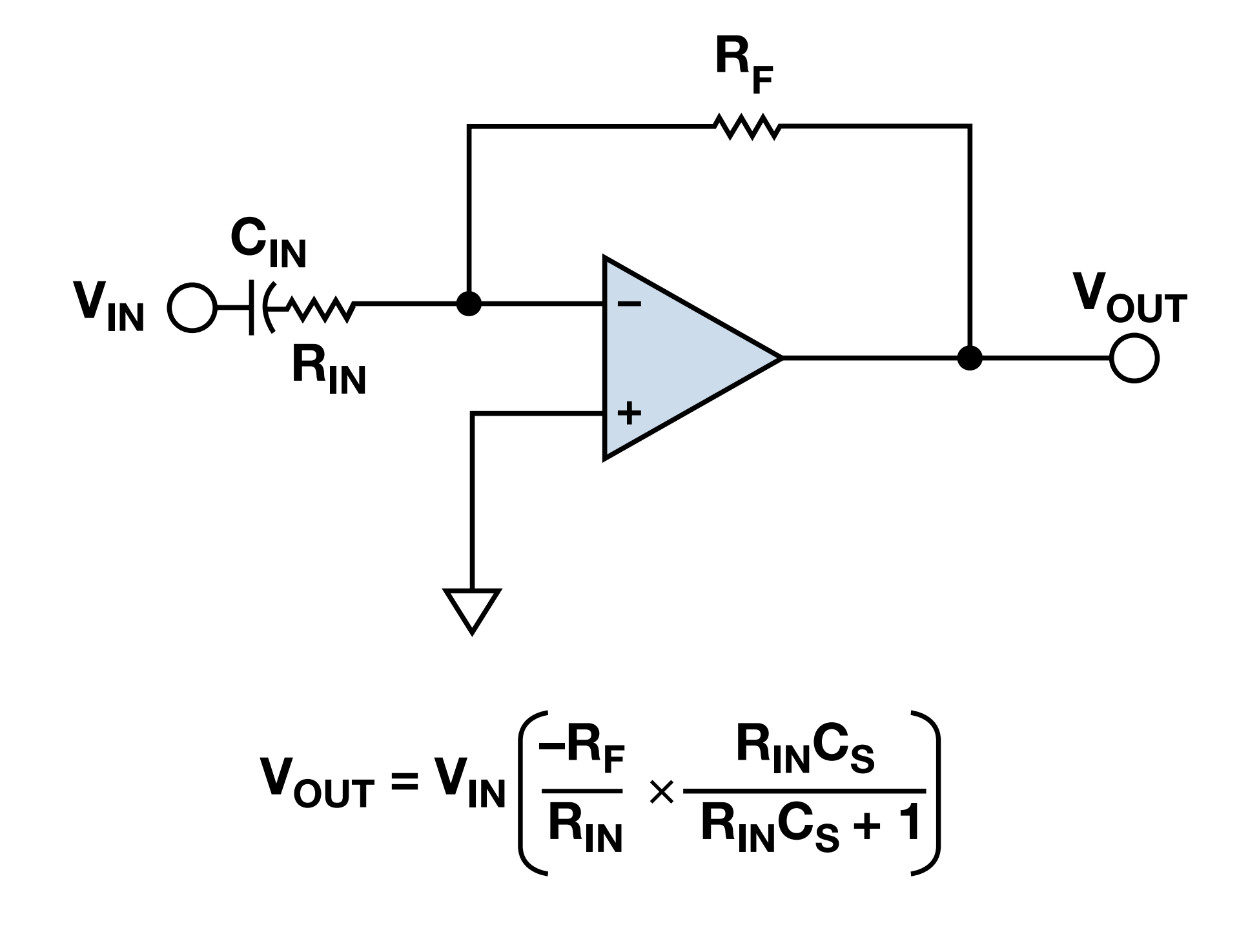### 差分放大器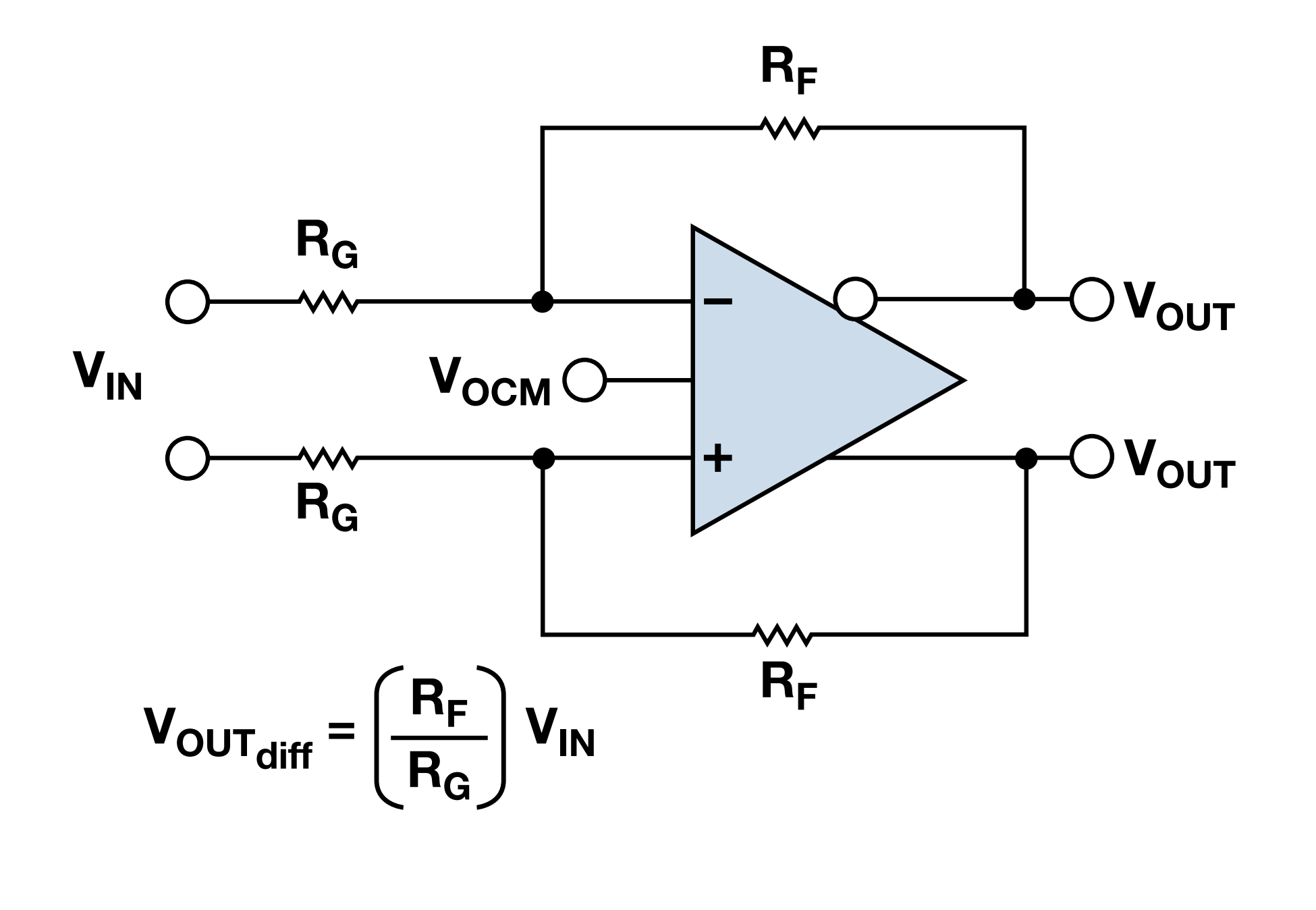### 仪表放大器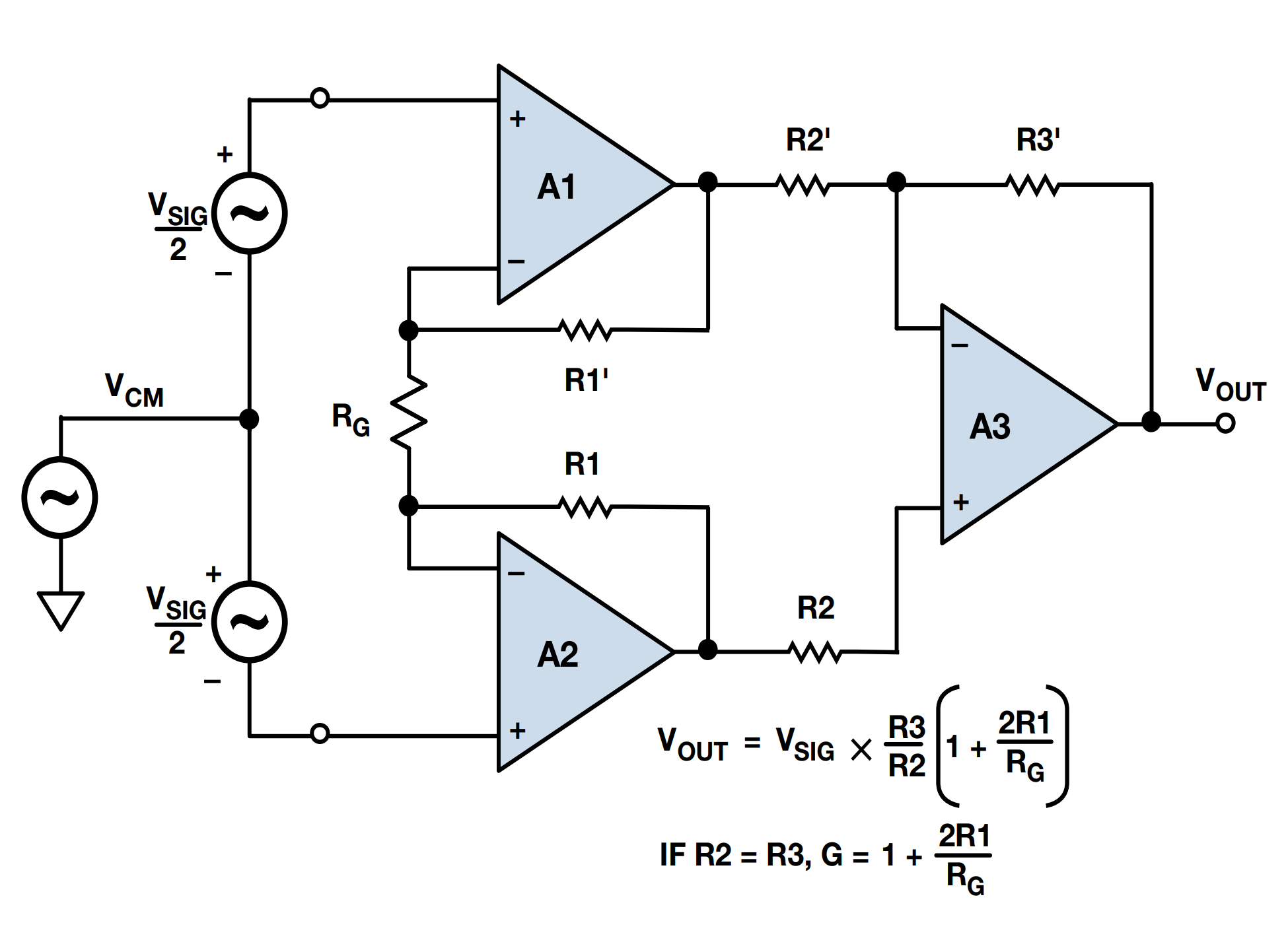## 运放的参数

### 输入失调电流

$I_{OS}=I_{B+}+I_{B-}$

### 输入偏置电流

$I_B=\frac{I_{B+}+I_{B-}}{2}$

### 增益带宽积

$GBW=A_{uo}*BW$

### 共模抑制比

$CMRR=20log(\frac{CMVR}{V_{O_{OS}}})$

### 转换速度

$SR=2 \pi f V_{pk}$

### 其他参数

• 共模电压范围 $$CMVR$$：也称为输入电压范围，如果两个输入端输入电压超出此范围，输出将发生削波或过大非线性现象。
• 全功率带宽：指在单位增益下测得的最大频率，在此频率下可以得到一个正弦信号的额定输出电压，且压摆率不会导致信号失真。
• 工作电源电压范围：运放正常工作时，能施加的电源电压范围。
• 电源抑制比 $$PSRR$$：电源电压的变化与输入失调电压的变化之比，结果用 dB 表示。
• 建立时间：施加一个阶跃输入后，放大器建立至某一预定的精度水平或输出电压百分比所需的时间。
• 电源电流：放大器空载工作时电源电压需提供的电流。

## 根据参数选型

1. 判断输入信号类型：直流需注意失调电流、失调电压；差分输入需判断是否选择仪表放大器；高频交流信号需注意增益带宽积 $$GBW$$ 和转换速度 $$SR$$
2. 判断精度要求：需要考虑失调电压、偏置电流、失调电流、共模抑制比对精度影响，判断是否选用高阻运放或精密运放。
3. 判断环境条件：需要注意运放的温度量程，注意温漂，注意电源纹波抑制比 $$PSRR$$ 的影响。
4. 判断其他要求：通道数、单 / 双电源供电（轨对轨信号失真小，可满幅值输出）、功率大小（高压 / 大电流情况下）。

### 根据用途选型

• 通用运放：对各类要求均不高的器件，注重通用与性价比。
• 音频运放：超低噪声（高保真）、低功耗（高续航）。
• 高速运放（$$GBW ≥ 50 MHz$$）：低功耗、低噪声 SNR。
• 功率运放：高电压、大电流。
• 精密运放（$$V_{os} < 1mV$$）：低失调电压，或低温漂、低噪声、低功耗、宽带宽。